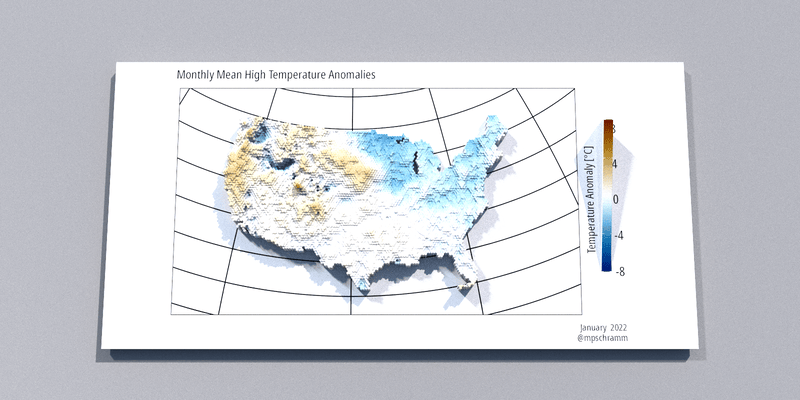# Drought condition gifs

Playing with drought maps.

Michael Schramm https://michaelpaulschramm.com (Texas Water Resources Institute)https://twri.tamu.edu
2022-08-18

I wanted to make a little gif showing monthly temperature anomalies for the year using PRISM data and rayshader.

Show code
``````## download monthly normals
get_prism_normals(type = "tmax",
resolution = "4km",
mon = 1:7,
keepZip = FALSE)
get_prism_monthlys(type = "tmax",
year = 2022,
mon = 1:7,
keepZip = FALSE)
## return the folders
tmean_norm_1 <- prism_archive_subset(type = "tmax",
temp_period = "monthly normals",
mon = 1:7,
resolution = "4km")
tmean_1 <- prism_archive_subset(type = "tmax",
temp_period = "monthly",
mon = 1:7)
## right now some are provisional and some are stable, so I need to reorder the vector
tmean_1 <- tmean_1[order(c(2,3,4,5,6,7,1))]

## convert to terra
tmean_norm_1 <- pd_to_file(tmean_norm_1)
tmean_norm_1 <- rast(tmean_norm_1)

tmean_1 <- pd_to_file(tmean_1)
tmean_1 <- rast(tmean_1)

## change the layer names to something sensible
names(tmean_norm_1) <- month.name[1:7]
names(tmean_1) <- month.name[1:7]

tanomaly <- tmean_1 - tmean_norm_1

tanomaly <- project(tanomaly, "epsg:2163")

## create and extent polygon
prism_ext <- as.polygons(ext(tanomaly), crs=crs(tanomaly))
prism_ext <- st_as_sf(prism_ext)

## create a hexagon grid in the extent polygon
hexagons <- st_make_grid(prism_ext,
n = c(150,150),
square = FALSE,
crs = crs(prism_ext))

## convert from sf back to terra,
## we lose the crs def along the way
## so set the crs again
hexagons <- vect(hexagons)
crs(hexagons) <- crs(tanomaly)

## calculate the mean raster values in each polygon
prism_summary_1 <- extract(x = tanomaly,
y = hexagons,
fun = mean,
na.rm = TRUE)

## extract returns a matrix, need to get the data back
## into hexagons
values(hexagons) <- data.frame(ID = 1:nrow(hexagons))
hexagons <- merge(hexagons, data.frame(prism_summary_1))
values(hexagons) <- data.frame(prism_summary_1)

ggplot() +
geom_spatvector(data = hexagons, aes(fill = January), color = NA) +
scale_fill_scico(name = "Temperature Anomaly [°C]", palette = "vik",
na.value = "transparent", limits = c(-5, 5)) +
guides(fill = guide_colorbar(title.position = "left",
barheight = 10)) +
theme_mps_noto() +
theme(legend.direction = "vertical",
legend.position = "right",
legend.title = element_text(angle = 90))

months <- month.name[1:7]
plots <- list()
for (i in 1:length(months)) {

fill <- noquote(months[i])
plots[[i]] <- ggplot() +
geom_spatvector(data = hexagons, aes_string(fill = fill), color = NA) +
scale_fill_scico(name = "Temperature Anomaly [°C]", palette = "vik",
na.value = "transparent", limits = c(-8, 8)) +
guides(fill = guide_colorbar(title.position = "left",
barheight = 10)) +
labs(subtitle = "Monthly Mean High Temperature Anomalies",
caption = paste(months[i], " 2022\n@mpschramm")) +
theme_mps_noto() +
theme(legend.direction = "vertical",
legend.position = "right",
legend.title = element_text(angle = 90),
axis.text = element_blank(),
axis.ticks = element_blank())

}

options(cores = 8)

for (i in 1:length(months)) {

plot_gg(plots[[i]],
width = 8,
height = 4,
solidcolor = "white",
theta = 0,
phi = 80,
fov = 45,
zoom = .5,
background = "grey80",
windowsize = c(1600, 800),
max_error = 0.01)

render_highquality(file = paste0("sp-rayshade-0", i, ".png"),
samples = 800,
sample_method = "sobol",
parallel = TRUE,
ground_material = rayrender::diffuse(color = "grey40",
sigma = 90),
environment_light = "dreifaltigkeitsberg_4k.hdr",
clamp_value = 5,
clear = TRUE)

}

library(gifski)

png_files <- sprintf("sp-rayshade-%s.png", c("01","02","03","04","05","06","07"))
gif_file <- gifski(png_files = png_files, width = 1600/2, height = 800/2, delay = 3)``````### Corrections

If you see mistakes or want to suggest changes, please create an issue on the source repository.

### Reuse

Text and figures are licensed under Creative Commons Attribution CC BY 4.0. Source code is available at https://github.com/mps9506/mschramm, unless otherwise noted. The figures that have been reused from other sources don't fall under this license and can be recognized by a note in their caption: "Figure from ...".

### Citation

For attribution, please cite this work as

`Schramm (2022, Aug. 18). @mpschramm: Drought condition gifs. Retrieved from https://michaelpaulschramm.com/posts/2022-08-18-drought/`

BibTeX citation

```@misc{schramm2022drought,
author = {Schramm, Michael},
title = {@mpschramm: Drought condition gifs},
url = {https://michaelpaulschramm.com/posts/2022-08-18-drought/},
year = {2022}
}```